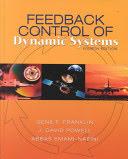### Automatic Control

Data is displayed for academic year: 2023./2024.

#### Course Description

Introducing course, terminology, historical overview. Classification of control systems. Principle of feedback. Formal representation of control systems. Mathematical modelling. Static and dynamic working regime. Linearization. Responses of linear time invariant (LTI) systems. Use of Laplace transform. Basic dynamic components of control systems. Transfer function and frequency characteristics. Stability analysis: algebraic and frequency methods. Performance indices of control systems. Introduction to design. PID regulator and parametrization of PID regulators. Windup and antiwindup.

#### Study Programmes

[FER3-EN] Electrical Engineering and Information Technology - study
(5. semester)

#### Learning Outcomes

1. explain the pricinple of feedback in control systems
2. apply laws of conservation of energy and matter in mathematical modelling of dynamical systems; linearize nonlinear model
3. employ block agebra and Laplace transform in transfer function calculation
4. compute frequency characteristics of linear systems
5. apply methods of analysis of stability of linear continuous-time control systems in frequency domain
6. apply tuning rules for PID regulator based on experiments (Ziegler-Nichols).

#### Forms of Teaching

Lectures

Two times per week two hours of lectures.

Exercises

One hour per week.

Independent assignments

6 homework assignments as preparation for laboratory exercises.

Laboratory

Laboratory exercises consist of 6 exercises. Each exercise is worth 3 points: 1 point for homework which is evaluated during the exercise, 0.5 for the laboratory work and 1.5 for a quiz written at the end of the exercise.

Continuous Assessment Exam
Laboratory Exercises 0 % 6 % 0 % 3 %
Homeworks 0 % 6 % 0 % 3 %
Quizzes 0 % 6 % 0 % 3 %
Mid Term Exam: Written 0 % 35 % 0 %
Final Exam: Written 0 % 47 %
Exam: Written 0 % 41 %
Exam: Oral 50 %
##### Comment:

Student must complete all laboratory exercises . On midterm exam and final exam at least 40 out of total 82 points is required. At least 50 out of total 100 points is required to pass the course.

#### Week by Week Schedule

1. Historical overview of control systems, Motivation for using control systems, Examples of control systems, Classification of control systems
2. Block diagrams and algebra , State space representation of continuous control systems , Modeling of mechanical systems
3. Modeling of electrical systems, Modeling of heath transfer processes, Modeling of fluid motion and accumulation, Linearization
4. Transfer function of continuous control systems , Transient and impulse system response, Forced and natural system response, Convolution integral
5. Pole and zero influence on LTI system time response
6. Control system quality criteria in time domain, Control error in steady-state
7. Closed loop control structures, Frequency characteristic
8. Midterm exam
9. Frequency characteristic, Nyquist diagram
10. Nyquist diagram, Bode diagram
11. Stability of LTI systems, Stability from transfer function, Algebraic stability criteria (Hurwitz)
12. Gain and phase margin, Graphic stability criteria (Nyquist and Bode)
13. PID controller structures, Tuning of PID controller parameters; Ziegler-Nichols methods
14. Tuning of PID controller parameters; Ziegler-Nichols methods, Practical aspects of PID controller design; Filtering; Anti-windup
15. Final exam

#### Literature

N. Perić, Z. Vukić, M. Baotić, M. Vašak, N. Mišković (2010.), Automatsko upravljanje - predavanja, Sveučilište u Zagrebu Fakultet elektrotehnike i računarstva
Z. Vukić, Lj. Kuljača (2005.), Automatsko upravljanje - analiza linearnih sustava upravljanja, Kigen
N. Mišković, T. Hadjina, N. Hure, M. Baotić (2015.), Automatsko upravljanje - zbirka zadataka, Sveučilište u Zagrebu Fakultet elektrotehnike i računarstvaG.F. Franklin, J.D. Powell, A. Emami-Naeini (2002.), Feedback Control of Dynamical Systems, Prentice Hall; 4th edition

#### General

ID 209745
Winter semester
5 ECTS
L1 English Level
L1 e-Learning
60 Lectures
0 Seminar
15 Exercises
12 Laboratory exercises
0 Project laboratory